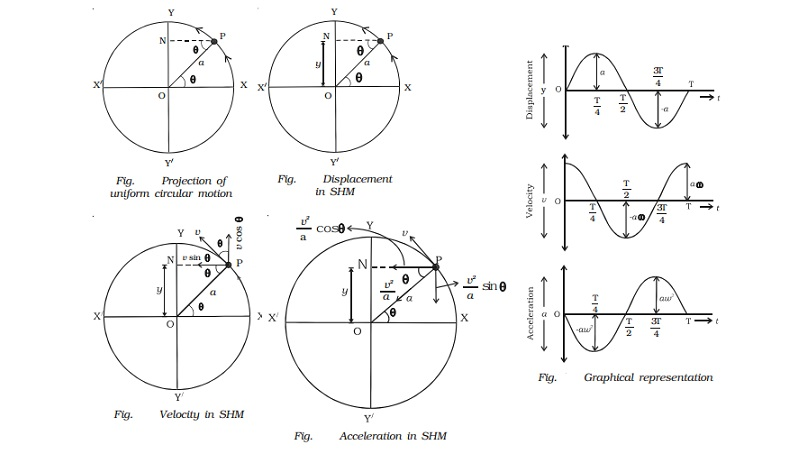Home | | Physics | | Physics | The projection of uniform circular motion on a diameter is SHM

# The projection of uniform circular motion on a diameter is SHMConsider a particle moving along the Y circumference of a circle of radius a and N P centre O, with uniform speed v, in anticlockwise direction as shown in Fig..

The projection of uniform circular motion on a diameter is SHM

Consider a particle moving along the Y circumference of a circle of radius a and N P centre  O,  with   uniform       speed v, in anticlockwise direction as shown in Fig..Let XX? and YY? be the two perpendicular X diameters.

Suppose the particle is at P after a time t. If ω is the angular velocity, then the angular displacement θ in time t is given by θ = ωt.

From P draw PN perpendicular to YY ? . As the particle moves from X to Y, foot of the perpendicular N moves from O to Y. As it moves further from Y to X ?, then from X ? to Y ? and back again to X, the point N moves from Y to O, from O to Y and back again to O. When the particle completes one revolution along the circumference, the point N completes one vibration about the mean position O. The motion of the point N along the diameter YY ? is simple harmonic.

Hence, the projection of a uniform circular motion on a diameter of a circle is simple harmonic motion.

Displacement in SHM

The distance travelled by the vibrating particle at any instant of time t from its mean position is known as displacement. When the particle is at P, the displacement of the particle along Y axis is y (Fig.).Then, in OPN, sin θ = ON/OP

ON = y = OP sin θ

y = OP sin ωt        ( θ = ωt)

since OP = a, the radius of the circle, the displacement of the vibrating particle is

y = a sin ωt  ?. (1)

The amplitude of the vibrating particle is defined as its maximum displacement from the mean position.

Velocity in SHM

The rate of change of displacement is the velocity of the vibrating particle.

Differentiating eqn. (1) with respect to time t

Dy/dt = d/dt (a sin ωt)

v = a ω cos ωt ...(2)

The velocity v of the particle moving along the circle can also be obtained by resolving it into two components as  hown in Fig..(i)                v cos θ in a direction parallel to OY

(ii)             v sin θ in a direction perpendicular to OY The component v sin θ has no effect along YOY ′ since it is perpendicular to OY.

Velocity = v cos θ   = = v cos ωt

We know that, linear velocity = radius ? angular velocity

v = aω

Velocity = aω cos ωt

Velocity = aω root(1-sin2 ωt)

Velocity = ω. Root(a2-y2)

Special cases

(i)                When the particle is at mean position, (i.e) y = 0. Velocity is aω and is maximum. v = aω is called velocity amplitude.

(ii)             When the particle is in the extreme position, (i.e) y = + a, the velocity is zero.

Acceleration in SHM

The rate of change of velocity is the acceleration of the vibrating particle.

D2y/dt2 = d/dt(dy/dt) = d/dt(( aω cos ωt)

= −ω2 a sin ωt

acceleration = d2y/dt2 = ?ω2 y   ??(4)

The acceleration of the particle can also be obtained by component method.

The centripetal acceleration of the particle P acting along P O is V2/a.This acceleration is resolved into two components as shown in Fig.

(i)                V2/a cos θ along P N perpendicular to OY

(ii)             V2/a sin θ in a direction parallal to YO.

The component v2/a  cos θ has no effect along YOY ′ since it is perpendicular to OY.

Hence acceleration = ? v2/a  . sin θ

= ? a ω2 sin ωt

= − ω2 y

acceleation = − ω2 y

The negative sign indicates that the acceleration is always opposite to the direction of displacement and is directed towards the centre.

Special Cases

When the particle is at the mean position (i.e) y = 0, the acceleration is zero.

When the particle is at the extreme position (i.e) y = +a, acceleration is a ω2 which is called as acceleration amplitude.The differential equation of simple harmonic motion from eqn. (4) is

D2y/dt2 + + ω2 y = 0

Using the above equations, the values of displacement, velocity and acceleration for the SHM are given in the Table 6.1. It will be clear from the above, that at the mean position y = 0, velocity of the particle is maximum but acceleration is zero. At extreme position y = +a, the velocity is zero but the acceleration is maximum a ω2 acting in the opposite direction.

Graphical representation of SHM

Graphical representation of displacement, velocity and acceleration of a particle vibrating simple harmonically with respect to time t is shown in Fig..

(i)                Displacement graph is a sine curve. Maximum displacement of the particle is y = +a.

(ii)             The velocity of the vibrating particle is maximum at the mean position i.e v = + a ω and it is zero at the extreme position.

(iii)           The acceleration of the vibrating particle is zero at the mean position and maximum at the extreme position (i.e) a ω2.

The velocity is ahead of displacement by a phase angle of  π/2  . The acceleration is ahead of the velocity by a phase angle π/2 or by a 2phase π ahead of displacement. (i.e) when the displacement has its greatest positive value, acceleration has its negative maximum value or vice versa.Study Material, Lecturing Notes, Assignment, Reference, Wiki description explanation, brief detail

Related Topics